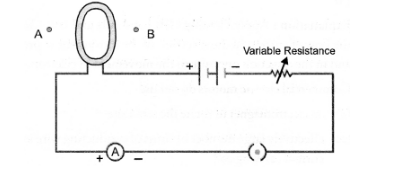# A circular loop placed in a plane perpendicular

Question:

A circular loop placed in a plane perpendicular to the plane of paper carries a current when the key is ON. The current is seen from points A and B (in

the plane of the paper and on the axis of the coil) is anticlockwise and clockwise respectively. The magnetic field lines point from B to A. The N-pole of

the resultant magnet is on the face close to

(a) A

(b) B

(c) A if current is small, and B if current is large.

(d) B if the current is small, and A if current is large.Solution:

(a).

Explanation : If current seems to flow in anticlockwise direction in a coil, then that face of the coil acts as N-pole.